Need Help?

Subscribe to Circuit

###### \${selected_topic_name}
• Notes
• Comments & Questions

$\begin{array}{l}{\text { Find the Norton equivalent circuit with respect }} \\ {\text { to the terminals } a, b \text { for the circuit shown. }}\end{array}$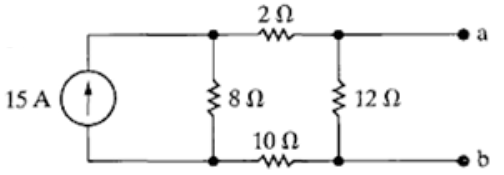Nortan الخطوات

(1) $S.C$ بين النقطتين

(2) $R_{th}=R_{ N}$

(3)(4) Norton نرسم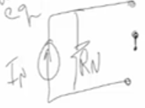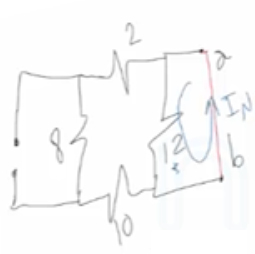$R_{eq}=2+8+10=20 \Omega$

$R_{eq}=\frac{20*12}{20+12}=75$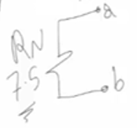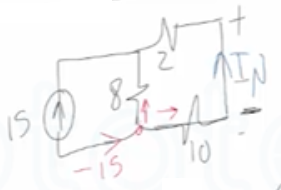$I_{N}=-15 * \frac{8}{8+2+10}=-6 A$

$\text { Norton }_{eq}$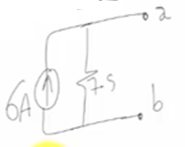No comments yet